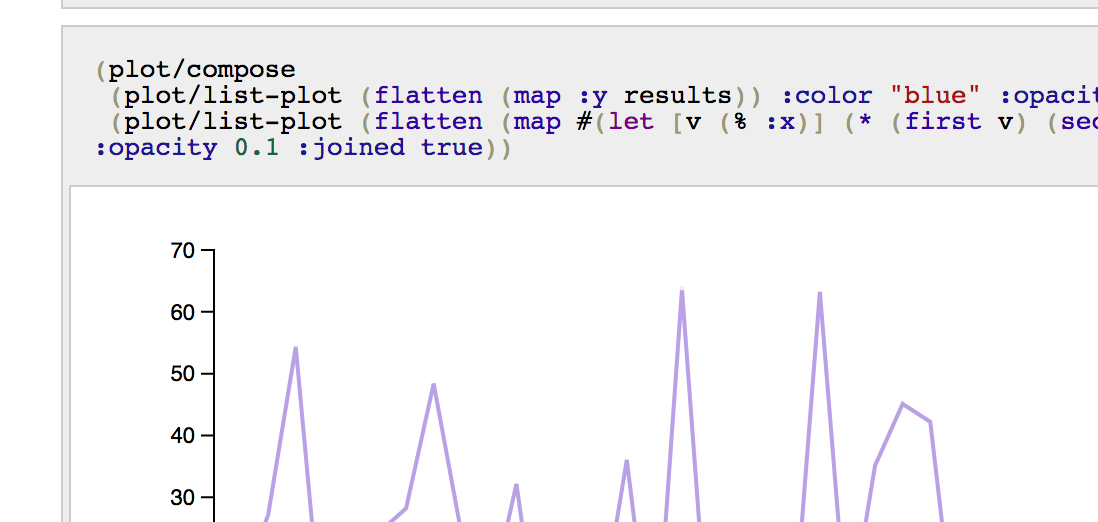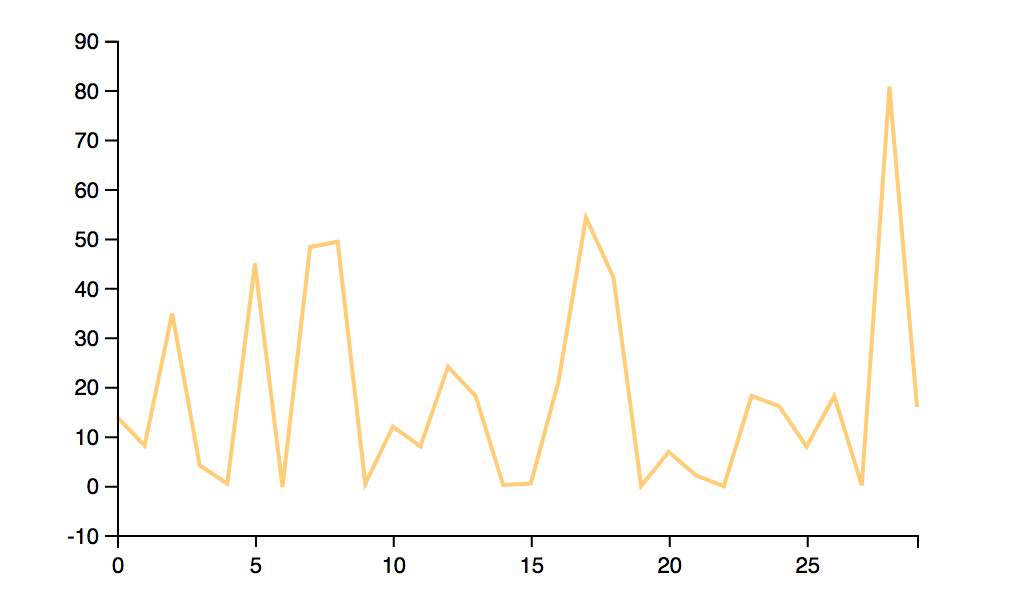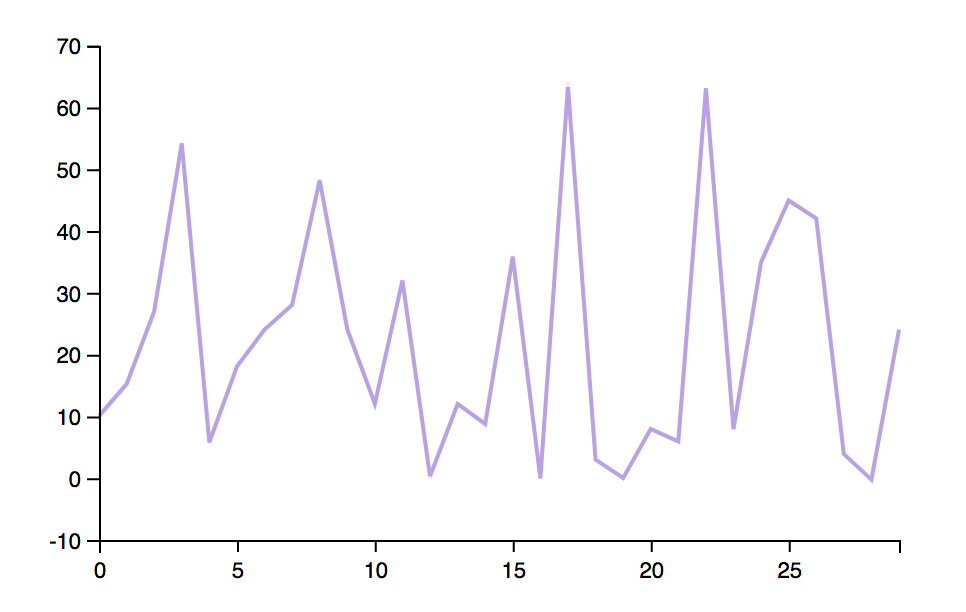Over a million developers have joined DZone.{{announcement.body}}
{{announcement.title}}

# Learning Machine Learning With Clojure and Cortex

DZone 's Guide to

# Learning Machine Learning With Clojure and Cortex

### Learn how to create easy-to-understand and ready-to-use neural networks from scratch and get instant results from your trained network using the Gorilla REPL.

· AI Zone ·
Free Resource

Comment (3)

Save
{{ articles.views | formatCount}} Views

It's not always easy to start something new. Machine learning is one of those programming skills that you may need to learn soon to go live with a new project but you don't really know where to start with it. Of course, you have already heard the hype, and you know the skill should be on your resume, but you never really got started.

While some of the simple tutorials are in Python, this article will use Clojure and Cortex for machine learning. You will learn how to create easy-to-understand and ready-to-use neural networks from scratch, as well as be able to get instant results from your trained network using the REPL dear to LISP.

Cortex is new, but it's a very strong alternative to existing machine learning frameworks. The fact that it is Clojure-based removes most of the surrounding boilerplate code required to train and run your own network.

Some examples I have created in the past with Cortex were used to classify cats and dogs or classify a large set of fruits. Both examples present how to write a neural network for image classification, but with this entry, we would like to go to the root of things and show you how to write an even simpler, but still effective, neural network — in just a few lines.

To highlight how to train and use the network, we will create a simple secret function (secret to the network, that is) and we will train the network to be able to compute inputs it has never seen before, quickly getting good results.

Cortex itself is a Clojure library that provides APIs to create and train your own network, including customizing input, output, and hidden layers, along with having an estimate of how good or bad the current trained network is.

The minimal Clojure project setup is a quite standard Leiningen setup, Leiningen being the de facto build tool for Clojure and a breeze to install. We will make use of the library `thinktopic/experiment` which is a high-level package of the Cortex library.We will also use one of my favorite REPLs, the Gorilla REPL, to have a Web REPL along plotting functions, which we will use later on.

``````(defproject cortex-tutorial "0.1-SNAPSHOT"
:plugins [[lein-gorilla "0.4.0"]]
:aliases {"notebook" ["gorilla" ":ip" "0.0.0.0" ":port" "10001"]}
:dependencies [
[org.clojure/clojure "1.8.0"]
[thinktopic/experiment "0.9.22"]])``````

The Gorilla plugin allows you to run a Web REPL, and you can start it by using the `notebook`  alias provided in the above `project.clj` file. Here's how it looks as a simple terminal or console command:

``lein notebook``

You are all set up and ready to get going. In a Clojure namespace, you are going to define three things:

1. The secret function that the network is supposed to map properly.

2. A generator for a sequence of random inputs.

3. A dataset generator to provide the network for training. This will call the `secret-fn` to generate both the input and output required to train the network.

In this project, the Clojure namespace containing the code is defined in `src/tutorial.clj` and will be used by the two Gorilla notebooks.

``````(ns tutorial)
(defn my-secret-fn [ [x y] ]
[ (* x y)])

(defn gen-random-seq-input []
(repeatedly (fn[] [(rand-int 10) (rand-int 10)] )))

(defn gen-random-seq []
(let[ random-input (gen-random-seq-input)]
(map #(hash-map :x % :y (my-secret-fn %)) random-input)))``````

With the Gorilla REPL started, head to the following local URL:

``http://127.0.0.1:10001/worksheet.html?filename=notebooks/create.clj``

This is where the REPL is located, and where you can follow along with the notebook and type Clojure code and commands directly into the browser.

## Preparation

The first task is to import some Cortex namespaces.

``````(ns frightened-resonance
(:require
[cortex.experiment.train :as train]
[cortex.nn.execute :as execute]
[cortex.nn.layers :as layers]
[cortex.nn.network :as network]

The network and layers namespaces will be used to define the internals of your network. The `train` namespace takes the network definition and datasets to produce a trained network. Finally, the `execute` namespace takes the trained network and an extra input-only dataset to run the network with the provided input. The `tutorial` namespace contains the code written above, with the hidden function and dataset generators.

Creating and testing the input generators will be your first step. The input generator produces a number of tuples made of two elements each.

``````(into [] (take 5 (tut/gen-random-seq-input)))
; [[9 0] [0 4] [2 5] [5 9] [3 9]]``````

The `random-seq` generator can provide datasets with both input and output, internally using the hidden function.

``````(into [] (take 5 (tut/gen-random-seq)))
; [{:y , :x [1 3]} {:y , :x [3 2]} {:y , :x [0 2]}{:y , :x [3 5]} {:y , :x [6 5]}]``````

Now that you have an idea of what the generated data looks like, let's create two datasets: both of 20,000 elements. `teach-dataset` will be used to tell the network what is known to be true and should remember what is true, while `test-dataset` will be used to indeed test the correctness of the network and compute its score. It is usually better to have two completely different sets.

``````(def teach-dataset
(into [] (take 20000 (tut/gen-random-seq))))

(def test-dataset
(into [] (take 20000 (tut/gen-random-seq))))``````

We have two strong, fantastic datasets, so let's write your network. The network will be defined as a common linear network made of four layers.

Two layers will be for the expected input and output, while two other layers will define the internal structure. Defining the layers of a neural network is quite an art in itself. Here, we take the hyperbolic tangent as the activation function. I actually got a better-trained network by having two -based activation layers.

The first layer defines the entrance of the network and its input, and says that there are two elements as one input, and that the label of the input is named `:x`.

The last layer defines the output of the network and says there is only one element and whose ID will be `:y`.

Using the Cortex API gives the small network code below:

``````(def my-network (network/linear-network
[(layers/input 2 1 1 :id :x)
(layers/linear->tanh 10)
(layers/tanh)
(layers/linear 1 :id :y)]))``````

All the blocks required to train the network are defined, so, as the Queen would say:

It's all to do with the training: you can do a lot if you're properly trained. — Queen Elizabeth II

## Training

The goal of training is to have your own trained network that you can either use right away or give to other users so that they can use your network completely standalone.

Training is done in steps. Each step takes elements of the teaching dataset in the batch and slowly fits the blocks of each layer with some coefficients so that the overall set of layers can give a result close to the expected output. The activation functions we are using are in some sense mimicing the human memory process.

After each teaching step, the network is tested for its accuracy using the provided test dataset. At this stage, the network is run with the current internal cofficients and compared with a previous version of itself to know whether it performs better or not, computing something known as the loss of the network.

If the network is found to be better than its last iteration, Cortex then saves the network as a NIPPY file, which is a compressed version of the network represented as a map. Enough said; let's finally get started with that training.

``````(def trained  (binding [*out* (clojure.java.io/writer "my-training.log")]
(train/train-n my-network teach-dataset test-dataset
:batch-size 1000
:network-filestem "my-fn"
:epoch-count 3000)))``````

The output of the training will be in the log file, and if you look, the first thing you can see in the logs is how the network is internally represented. Here are the different layers, with the input and output sizes for each layer and the number of parameters to fit.

``````Training network:
| type | input | output | :bias | :weights |
|---------+-------------+-------------+-------+----------|
| :linear | 1x1x2 - 2 | 1x1x10 - 10 |  | [10 2] |
| :tanh | 1x1x10 - 10 | 1x1x10 - 10 | | |
| :tanh | 1x1x10 - 10 | 1x1x10 - 10 | | |
| :linear | 1x1x10 - 10 | 1x1x1 - 1 |  | [1 10]
|Parameter count: 41``````

Then, each step/epoch gets the new score sees and whether the network was better and, in that case, saved.

``````| :type | :value | :lambda | :node-id | :argument |
|-----------+-------------------+---------+----------+-----------|
| :mse-loss | 796.6816681755391 | 1.0 | :y |
|Loss for epoch 1: (current) 796.6816681755391 (best) nullSaving network to my-fn.nippy``````

The score of each step gives the effectiveness of the network, and the closer the loss is to zero, the better the network is performing. So, while training your network, one of your goals should be to get that loss value as close to zero as possible.

The full training with 3,000 epochs should only take a few minutes, and once it's done, you can immediately find out how the trained network is performing. If you are in a hurry, 1,500-2,000 is a good range for the number of epochs that will give you a compromise between speed and an already quite accurated trained network.

Once the training is done, you will find a new `my-fn.nippy` file in the current folder. This is a compressed file based on the version of the trained cortex network.

A copy of the trained network, `mynetwork.nippy`, has been included in the companion project. The loss of the network was pretty good, with a value very close to zero, as seen below.

``Loss for epoch 3000: (current) 0.031486213861731005 (best)0.03152873877808952Saving network to my-fn.nippy``

Let's give our newly trained network a shot, with a manually defined custom input.

``````(execute/run trained [{:x [0 1]}])
; [{:y [-0.09260749816894531]}]``````

This is quite close to the expected 0*1=0 output.

Now, let's try something that the network has never seen before with a tuple of double values.

``````(execute/run trained [{:x [5 1.5]}])
; [{:y [7.420461177825928]}]``````

Sweet. 7.42 is quite good compared to the expected result, 5*1.5=7.5.

## Using the Network

As you saw, the trained network was saved in a NIPPY file. That file can be loaded and used by external "users" of your network. From now on, if you look at the provided notebooks, you can load the following note:

``http://127.0.0.1:10001/worksheet.html?filename=notebooks/use.clj``

The users need a few namespaces. The `tutorial`  and `execute` namespaces you've seen (i.e. `util`) will be used to load the network from a file, and `plot` is provided by the Gorilla plugin to plot values.

Later, we plan to plot the expected results vs. the network provided results.

``````(ns sunset-cliff
(:require
[cortex.nn.execute :as execute]
[cortex.util :as util]
[tutorial :as tut]
[gorilla-plot.core :as plot]

Loading the trained network is a simple matter of using the `read-nippy-file` function provided by Cortex.

``(def trained (util/read-nippy-file "mynetwork.nippy"))``

We didn't look at it before, but the network is indeed a map, and you can check its top-level keys.

``````(keys trained)
; (:compute-graph :epoch-count :traversal :cv-loss)``````

It is a good idea to check the number of epochs that the network has gone through, along with its current loss value.

``````(select-keys trained [:epoch-count :cv-loss])
; {:epoch-count 3000, :cv-loss 0.030818421955475947}``````

You can confirm that the loaded network is given the same result as its freshly trained version from the last section.

``````(execute/run trained [{:x [5 1.5]}])
; [{:y [7.420461177825928]}]``````

Now, let's generate a bunch of results with the loaded network and plot them. (Running the network on a fresh input set is now trivial for you!)

``````(def input
(into [] (take 30 (tut/gen-random-seq))))
(def results (execute/run trained input))``````

And, of course, we can check a few of the result values produced by the network.

``(clojure.pprint/pprint (take 3 results))``

Plotting can be done with the Gorilla-provided plotting functions from the `gorilla-plot.core` namespace. Here, we will take interest only in the output, and we will use Clojure's `flatten` function to create a flat collection of output values as opposed to the sequence of vectors found in the results.

``(plot/list-plot (flatten (map :y results)) :color "#ffcc77" :joined true)``

After specifying a color, and knowing that `plot` should use lines instead of dots, you can see the graph below straight in the browser REPL.You can also produce a "composed" graph made of the expected results produced straight from the known or not-hidden function vs. results produced by the trained network.

``````(plot/compose
(plot/list-plot
(flatten (map :y results))
:color "blue"
:opacity 0.3
:joined true)
(plot/list-plot
(flatten (map #(let [v (% :x)] (* (first v) (second v))) input))
:color "blue"
:opacity 0.3
:joined true))``````

The two lines are actually too close and quite entirely overlap each other.## Retrain

From there, an interesting progression path would be to take the currently trained network and make it better by using more datasets and running the cortex `train-n` function again.

``````(require '[cortex.experiment.train :as train])
(def teach-dataset
(into [] (take 200 (tut/gen-random-seq))))

(def test-dataset
(into [] (take 200 (tut/gen-random-seq))))

(def re-trained
(binding [*out* (clojure.java.io/writer "re-train.log")]
(train/train-n trained teach-dataset test-dataset
:batch-size 10
:network-filestem "retrained"
:epoch-count 10)))``````

Note: By specifying a new `network-filestem`, you can keep a separate version of the updated network.

And with new datasets and a new training cycle, there is still a good chance to achieve a better network, and in that case, the network is saved again using the new file name.

``````Loss for epoch 3012: (current) 0.03035303473325996 (best)0.030818421955475947
Saving network to retrained.nippy``````

## Conclusion

You have seen in this post how to train your own neural network to simulate the output of a known function. You saw how to generate and provide the required datasets used for training and how Cortex saves a file version of the best network.

Now, some ideas would be to:

• Try the same for a different hidden function of your own.
• Change the number of input and output parameters of the function and the network in the network metadata.
• If the network does not perform as well as expected, spend some time on the different layers of the network and find a better configuration.
Topics:
clojure ,machine learning ,neural network ,ai ,tutorial ,gorilla repl ,cortex

Comment (3)

Save
{{ articles.views | formatCount}} Views

Published at DZone with permission of

Opinions expressed by DZone contributors are their own.